# Log-spherical Mapping in SDF Raymarching

Pierre Cusa <pierre@osar.fr>
February 2019 (history)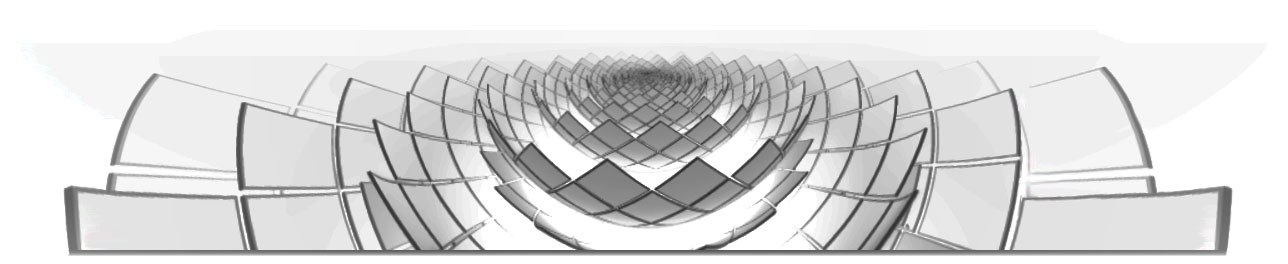In this post, I'll describe a set of techniques for manipulating signed distance fields (SDFs) allowing the creation of self-similar geometries like the flower above. Although these types of geometries have been known and renderable for a long time, I believe the techniques described here offer much unexplored creative potential, since they allow the creation of raymarchable SDFs of self-similar geometries with an infinite level of visual recursion, which can be explored in realtime on the average current-gen graphics card. This is done by crafting distance functions based on the log-spherical mapping, which I'll explain starting with basic tilings and building up to the recursive shell transformation.

## Log-polar Mapping in 2D

Conversions between log-polar coordinates $(\rho, \theta)$ and Cartesian coordinates $(x, y)$ are defined as:

$$\tag{1} \begin{cases} \rho = \log\sqrt{x^2 + y^2}, \cr \theta = \arctan\frac{y}{x}. \end{cases}$$ $$\tag{2} \begin{cases} x = e^\rho\cos\theta,\hphantom{\sqrt{x^2+}} \cr y = e^\rho\sin\theta. \end{cases}$$

The conversion $(2)$ can be used as an active transformation, the inverse log-polar map, in which we can consider $(\rho, \theta)$ as the Cartesian coordinates before the transformation, and $(x, y)$ after the transformation. Applying this to any geometry that regularly repeats along the $\rho$ axis results in a self-similar geometry. Let's apply it to a grid of polka dots to get an idea of how this happens.

We'll be implementing this in GLSL fragment shaders, which means everything needs to be reversed: instead of defining shapes and applying transformations to them, we first apply the inverse of those transformations to the pixel coordinates, then we define the shapes based on the transformed coordinates. This is all done in a few lines:

float logPolarPolka(vec2 pos) {
// Apply the forward log-polar map
pos = vec2(log(length(pos)), atan(pos.y, pos.x));

// Scale everything so tiles will fit nicely in the ]-pi,pi] interval
p *= 6.0/PI;

// Convert pos to single-tile coordinates
pos = fract(pos) - 0.5;

// Return color depending on whether we are inside or outside a disk
return 1.0 - smoothstep(0.3, 0.31, length(pos));
}


And here is the result in a complete shader with some extra controls: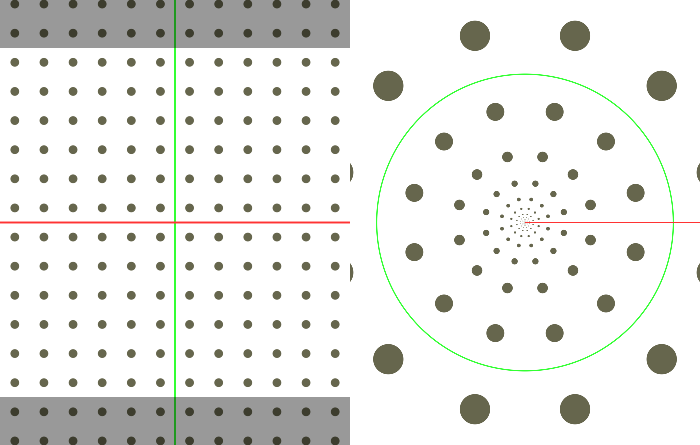Log-polar tiling in 2D. Controls perform translation before mapping. Red axis: $\rho$, green axis: $\theta$

Note how regular tiling yields self-similarity, $\rho$-translation yields scaling, and $\theta$-translation yields rotation. Also visible, the mapping function's domain does not cover the whole space, but is limited to $\theta\in\left]-\pi, \pi\right]$ as represented by the dark boundaries.

## Estimating the Distance

The above mapping function works well when applied to an implicit surface, but in order to prepare for 3D raymarching, we should apply it to a distance field, and obtain an equally correct distance field. Unfortunately, the field we obtain is heavily distorted as visualized below on the left side: the distance values are no longer correct since they are shrunk along with the surface. In order to correct for this, consider that the mapping effectively scales geometry by the radial distance $r=\sqrt{x^2 + y^2}$ (proof too large to fit in the margin). Furthermore, as Inigo Quilez notes, when scaling a distance field, we should multiply the distance value by the scaling factor. Thus the simplest correction is to multiply the distance by $r$. In most cases, this correction gives a sufficiently accurate field for raymarching.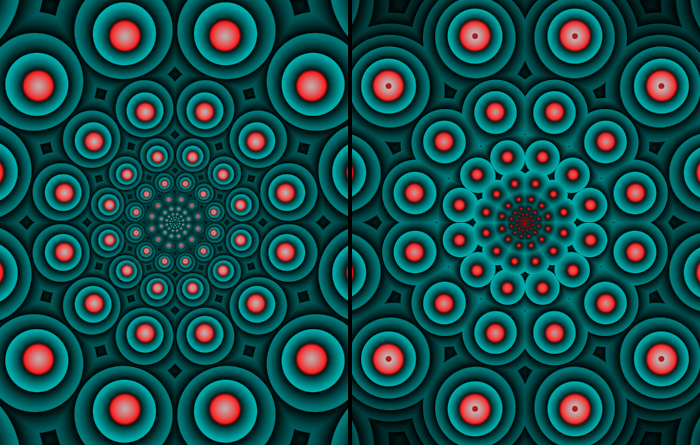Distance field correction. Left: distorted field, right: corrected field. Colors represent the distance field's contour lines, red: negative, blue: positive.

## Log-polar Mapping in 3D

The above 2D map can be simply applied in 3D space by picking two dimensions to transform. However, this means the geometry will get scaled in those two dimensions but not in the remaining dimension (in fact, geometry will be infinitely slim at the origin, and infinitely fat at the horizon). This is a problem because SDF scaling must be uniform, but we can easily correct this by scaling the remaining dimension proportionally to the others, using the same $r$ factor as before. If we're tiling spheres, this would give us the following distance function:

#define SCALE 6.0/PI

float sdf(in vec3 pos3d)
{
// Choose 2 dimensions and apply the forward log-polar map
vec2 pos2d = pos3d.xz;
float r = length(pos2d);
pos2d = vec2(log(r), atan(pos2d.y, pos2d.x));

// Scale pos2d so tiles will fit nicely in the ]-pi,pi] interval
pos2d *= SCALE;

// Convert pos2d to single-tile coordinates
pos2d = fract(pos2d) - 0.5;

// Get ball distance;
// Shrink Y coordinate proportionally to the other dimensions;
// Return distance value multiplied by the final scaling factor
float mul = r/SCALE;
return (length(vec3(pos2d, pos3d.y/mul)) - radius) * mul;
}


Distance functions made using this technique work well with raymarching: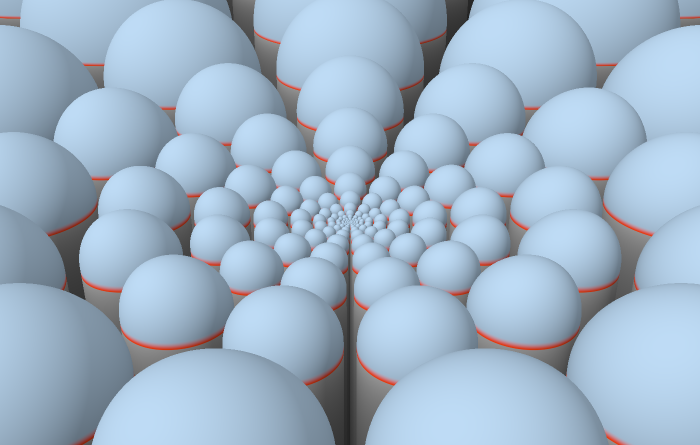Log-polar mapping applied to two dimensions of a 3D geometry.

## Log-spherical Mapping

The above technique lets us create, without loops, self-similar surfaces with an arbitrary level of detail. But the recursion still only happens in two dimensions - what if we want fully 3D self-similar geometries? Log-polar coordinate transformations can be generalized to more dimensions. Conversions between log-spherical coordinates $(\rho, \theta, \phi)$ and Cartesian coordinates $(x, y, z)$ are defined as:

$$\tag{3} \begin{cases} \rho = \log\sqrt{x^2 + y^2 + z^2}, \cr \theta = \arccos\frac{z}{\sqrt{x^2 + y^2 + z^2}} \cr \phi = \arctan\frac{y}{x}. \end{cases}$$ $$\tag{4} \begin{cases} x = e^\rho\sin\theta\cos\phi, \hphantom{\log x^2} \cr y = e^\rho\sin\theta\sin\phi, \cr z = e^\rho\cos\theta. \end{cases}$$

Again, conversion $(4)$ can be used as an active transformation. This is the inverse log-spherical map, which results in a self-similar geometry when applied to a regularly repeating geometry. $\rho$-translation now yields uniform scaling in all axes.Log-spherical mapped geometry.

## Similarity

With the above technique, any geometry we define will be warped into a spherical shape, with visible pinching at two poles. What if we want to create a self-similar version of any arbitrary geometry, without this deformation? For this, the mapping needs to be similar, that is, preserving angles and ratios between distances. To achieve this, we apply the forward log-spherical transformation before applying its inverse, and perform regular tiling on the intermediate geometry. The combined mapping function's domain is a spherical shell, inside which we can define any surface, and have it infinitely repeated inside and outside of itself - without relying on loops. This is implemented in the following distance function:

// pick any density and get its inverse:
float dens = 2.0;
float idens = 1.0/dens;

float sdf(in vec3 p)
{
// Apply the forward log-spherical map
float r = length(p);
p = vec3(log(r), acos(p.z / r), atan(p.y, p.x));

// find the scaling factor for the current tile
float scale = floor(p.x*dens)*idens;

// Turn tiled coordinates into single-tile coordinates
p.x = mod(p.x, idens);

// Apply the inverse log-spherical map
float erho = exp(p.x);
float sintheta = sin(p.y);
p = vec3(
erho * sintheta * cos(p.z),
erho * sintheta * sin(p.z),
erho * cos(p.y)
);

// Get distance to geometry in the prototype shell
float ret = shell_sdf(p);

// Correct distance value with the scaling applied
return ret * exp(scale);
}


We can test this by putting some boxes and cylinders in the prototype shell: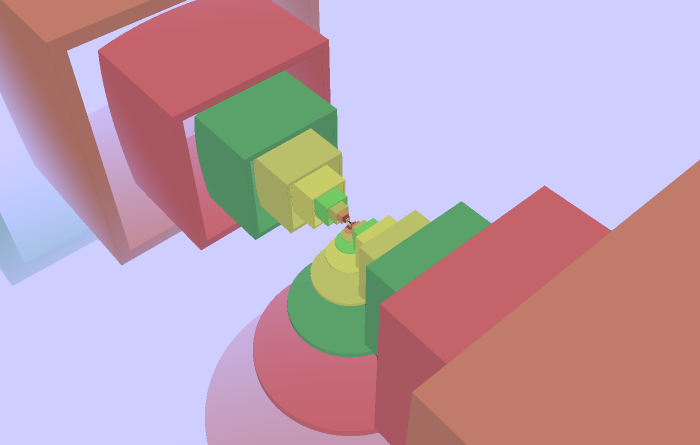Similar log-spherical mapped geometry.

## Implementation Challenges

Anyone experimenting with these techniques will inevitably run into problems due to distance field discontinuities, so it would be really scummy not to include a section on this topic. The symptoms will appear as visible holes when raymarching, as rays will sometimes overshoot the desired surface. To better understand the problem, the classic SDF debugging technique is to take a cross-section of a raymarched scene and to color it according to its distance value.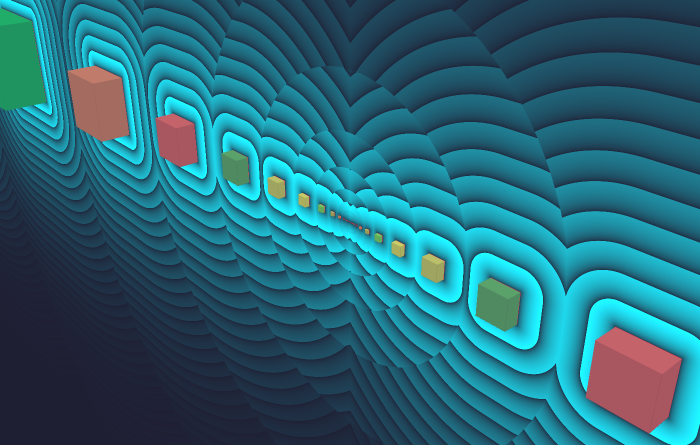Log-spherical scene with distance gradient shown in a cross-section.

Under the log-spherical mapping, repeated tiles become spherical shells contained inside of each other. Visualized above is the discontinuity in the distance gradient at the edges between shells, due to each tile only providing the distance to the geometry inside of it. Here, the "twist" parameter modifies the scene in a way that accentuates the discontinuities. This type of problem can be fixed in several ways, all of which are tradeoffs against rendering performance:

• Add hidden geometry to bring rays into the correct tile. In some cases, we know that the final geometry will approximately conform to a certain plane or other simple shape. We can estimate this shape's distance, cut off its field below a certain threshold so it's never actually hit, and combine it into the scene.
• Combine the SDF for adjacent tiles into each tile, so that the distance value takes into account more surrounding geometry. This is especially useful when the contents of each tile are close to, or touching each other.
• Shorten the raymarching steps. This can be done globally, or only locally in problematic regions.

## Examples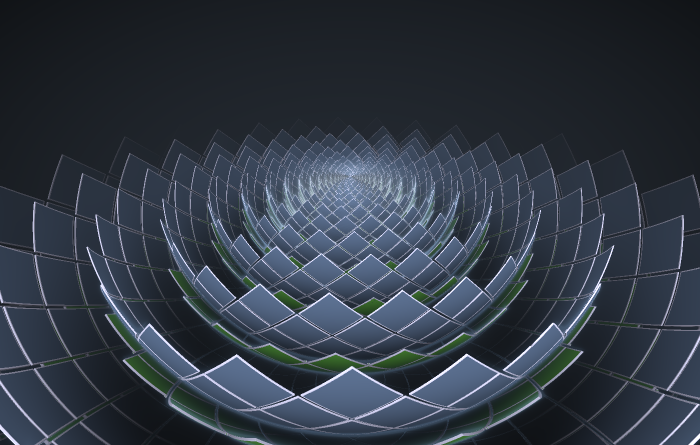Recursive Lotus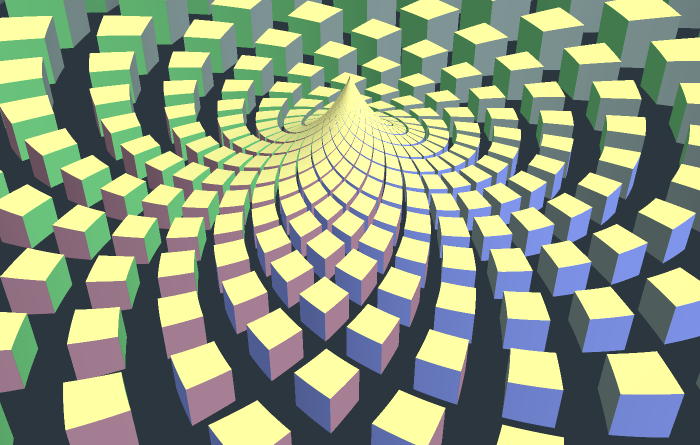Cube Singularity

## Prior Art, References and More

While I haven't found any previous utilization of the log-spherical transformation in shaders, there are many related and similar techniques that have been creatively used:

• The 2D log-polar transformation, and its related logarithmic spirals, have been used since the early days of fragment shader programming. They have also been developed into much more interesting and complex structures and related transformations. A nice example: Flexi's Bipolar Complex.
• Applying the 2D log-polar transform to 3D SDFs has been done previously, but apparently only as a cursory exploration, see knighty's Spiral tiling shader.
• Self-similar structures and zoomers have also been implemented in shaders using loops, as opposed to the loopless techniques described here. Looping strongly limits the number of visible levels of recursion for realtime, however, it allows more freedom in geometry placement while giving an exact distance. Some examples of this: Gimbal Harmonics; Infinite Christmas Tree, possibly Quite's zeo-x-s.
• Outside of SDF raymarching, self-similar structures have been explored in many different ways, a popular example being the works of John Edmark.

If you want to explore these techniques, or creative shader programming in general, Shadertoy is where a lot of the action happens these days. I've made an account where I'll make sure to post some more experiments with these techniques.